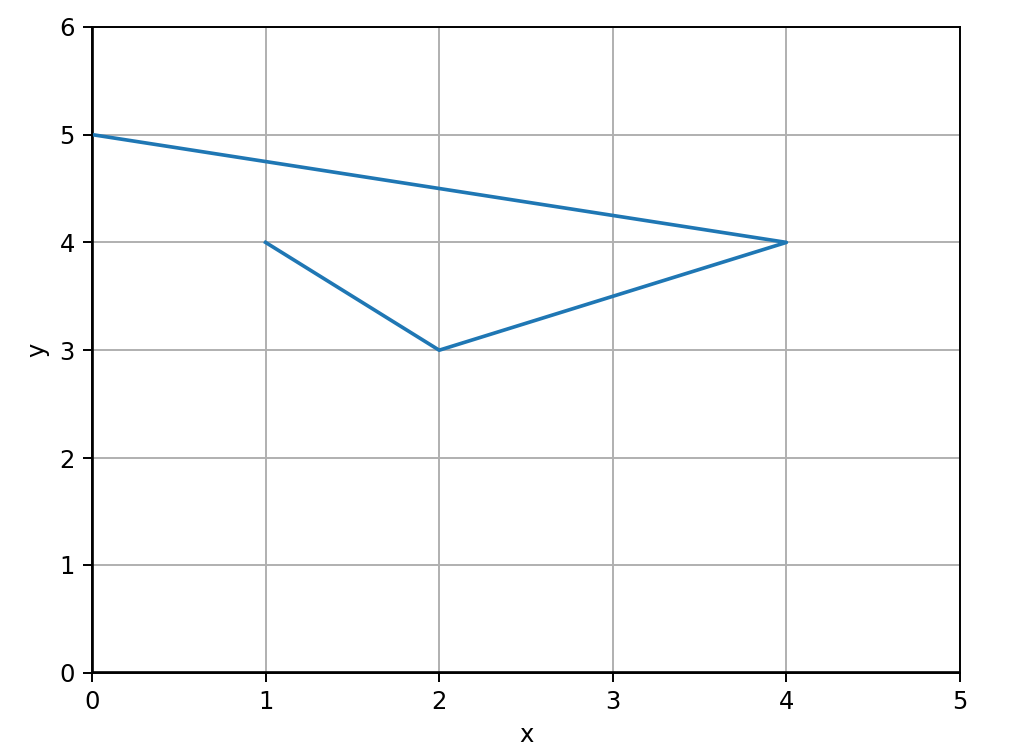# Learning Python with Advent of Code Walkthroughs

Dazbo's Advent of Code solutions, written in Python

# The Python Journey - Zip## Overview

The `zip()` function is very cool!

In short, it combines n iterables of length x, to create a single new iterable of length n, with each element being a tuple of n items.

What does THAT mean?

It’s easier with an example…

``````questions = ['Your name?', 'Your quest?', 'Your favourite color?']
answers = ['Lancelot', 'The Holy Grail', 'Blue. No... Red!']

# print the tuple
print(item)
``````

The output:

``````('Your name?', 'Lancelot')
('Your favourite color?', 'Blue. No... Red!')
``````

So here, we started with 2 lists that both had 3 elements. By zipping them together, we ended up with one list made up of tuples, with each tuple being made up of 2 elements.

## Iterating Over Two Iterables at Once

We can even do this:

``````questions = ['name', 'quest', 'favourite color']
answers = ['I am Arthur, King of the Britons', 'We seek the Holy Grail', 'Blue!']

# unpack and format the tuple
for q, a in zip(questions, answers):
``````

Output:

``````What is your name?  I am Arthur, King of the Britons
What is your quest?  We seek the Holy Grail
What is your favourite color?  Blue!
``````

## Transposing Rows and Columns

Imagine we have four different lists:

• One list containing all the days of the week.
• Three more lists, where each list contains a percentage change for a coin value on that day of the week.

We can think of this as four rows and seven columns. Using `zip()`, we can turn this into seven rows of four columns:

``````# daily % changes for these coins, over 6 days
day = ['Monday', 'Tuesday', 'Wednesday', 'Thursday', 'Friday', 'Saturday', 'Sunday']
btc = [3.4, 1.5, 5.2, -2, -3, 7.1, 8.0]
eth = [0.4, 0.2, 7.0, 2.5, 1.5, 8.0, 10.0]
doge = [-12.8, -30.0, -10.0, 0.0, 30.2, 11.1, 15.0]

for item in zip(day, btc, doge, eth):
print(item)
``````

Output:

``````('Monday', 3.4, -12.8, 0.4)
('Tuesday', 1.5, -30.0, 0.2)
('Wednesday', 5.2, -10.0, 7.0)
('Thursday', -2, 0.0, 2.5)
('Friday', -3, 30.2, 1.5)
('Saturday', 7.1, 11.1, 8.0)
('Sunday', 8.0, 15.0, 10.0)
``````

## Unpacking An Arbitrary Number of Iterables

We can use the splat operator to zip any arbitrary number of iterables. For example, we could re-write the example above like this:

``````# daily % changes for these coins, over 6 days
day = ['Monday', 'Tuesday', 'Wednesday', 'Thursday', 'Friday', 'Saturday', 'Sunday']
btc = [3.4, 1.5, 5.2, -2, -3, 7.1, 8.0]
eth = [0.4, 0.2, 7.0, 2.5, 1.5, 8.0, 10.0]
doge = [-12.8, -30.0, -10.0, 0.0, 30.2, 11.1, 15.0]

coin_changes = [day, btc, doge, eth]    # Making a single list of lists, to demonstrate splatting
# unpack (splat) the list of lists, and then zip the resulting lists
transposed = list(zip(*coin_changes))
for row in transposed:
print(row)
``````

## Turning (x,y) coordinates into a List of x and a List of y

Sometimes it can be really useful to extract all the x values and all the y values, from a collection of points. For example, if plotting multiple points with `matplotlib.pyplot.plot()`, the `plot()` method expects all the x values as an array, and all the y values as an array.

``````from matplotlib import pyplot as plt

# create list of points
points = [
(1, 4),
(2, 3),
(4, 4),
(0, 5)
]

print(points)

# Unpack our x, y vals
all_x, all_y = zip(*points)
print(f"all_x: {all_x}")
print(f"all_y: {all_y}")

# Create out axes
fig, axes = plt.subplots()

# add lines at x=0, y=0 and labels
plt.axhline(0, color='black')
plt.axvline(0, color='black')
axes.set_xlim(0, max(all_x)+1)
axes.set_ylim(0, max(all_y)+1)
axes.set_xlabel("x")
axes.set_ylabel("y")

axes.grid(True)

plt.plot(all_x, all_y)
plt.show()
``````

The output:

``````[(1, 4), (2, 3), (4, 4), (0, 5)]
all_x: (1, 2, 4, 0)
all_y: (4, 3, 4, 5)
``````

And the rendered plot:## Making a Mapping Dictionary

We can use `zip()` to create a `dictionary` that maps one value to another. For example:

``````openers = ["(", "[", "{", "<"]
closers = [")", "]", "}", ">"]
open_to_close = dict(zip(openers, closers))

print(open_to_close)
``````

Output:

``````{'(': ')', '[': ']', '{': '}', '<': '>'}
``````